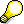CAPP Standard Value CalculationWhen creating or changing a routing, you can calculate standard values with CAPP. The system displays the calculated standard values in the Standard values section of the Operation Details screen. The value type assigned to the CAPP method for calculating determines the fields in which the calculated standard values are displayed. You can define value types in Customizing.The standard values of an operation can only be calculated with CAPP if there is at least one CAPP process assigned to the work center at which the operation is carried out.

Proceed as follows to calculate standard values:

1. On the operation overview, select the operations for which you want to calculate standard values.
2. Select the menu options Extras ® CAPP and specify whether you want the processes and methods to be selected manually or automatically by the system.

With automatic selection, the system selects the processes and methods which are marked with a standard indicator.

With manual selection, you make the selection. The system branches to the Sub-calculations screen which shows a list of all the processes assigned to the work center. Select the desired process and select the menu options Std val. calculation ® Next screen.

On the following screens, you can select a method and assign values to characteristics.

The results from the individual calculations are displayed within CAPP on the Method Result screen. The Sub - calculations screen shows the results of any calculations carried out for an operation.

If the standard values of an operation in a routing have been calculated using CAPP, on the Operation Details screen, the system displays the year when the calculation was carried out as well as a key which specifies that the values were calculated with CAPP.

For additional information about calculating standard values using CAPP, refer to the
PP CAPP Standard Value Calculation guide.